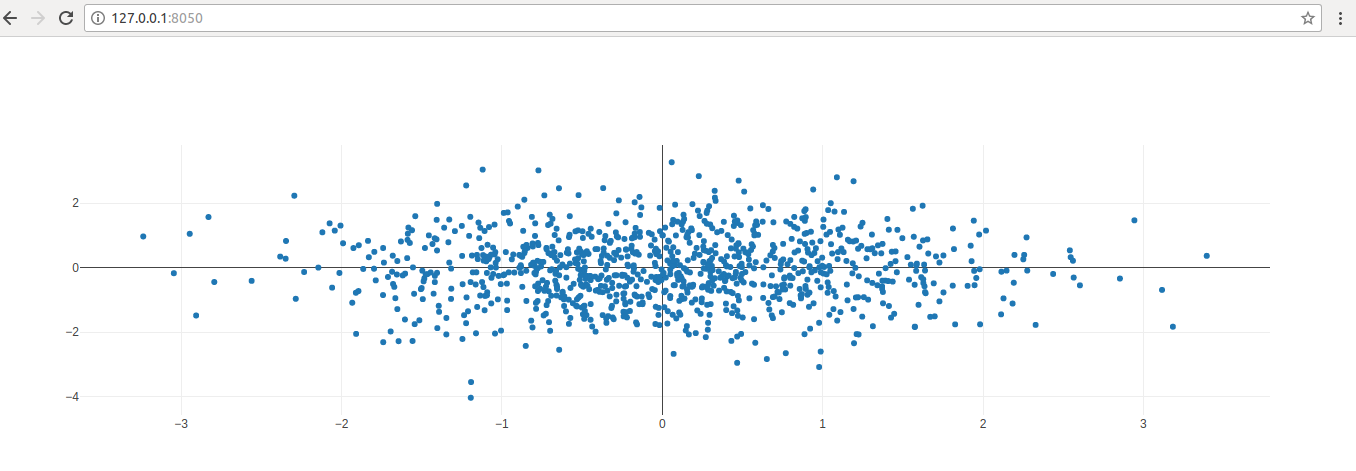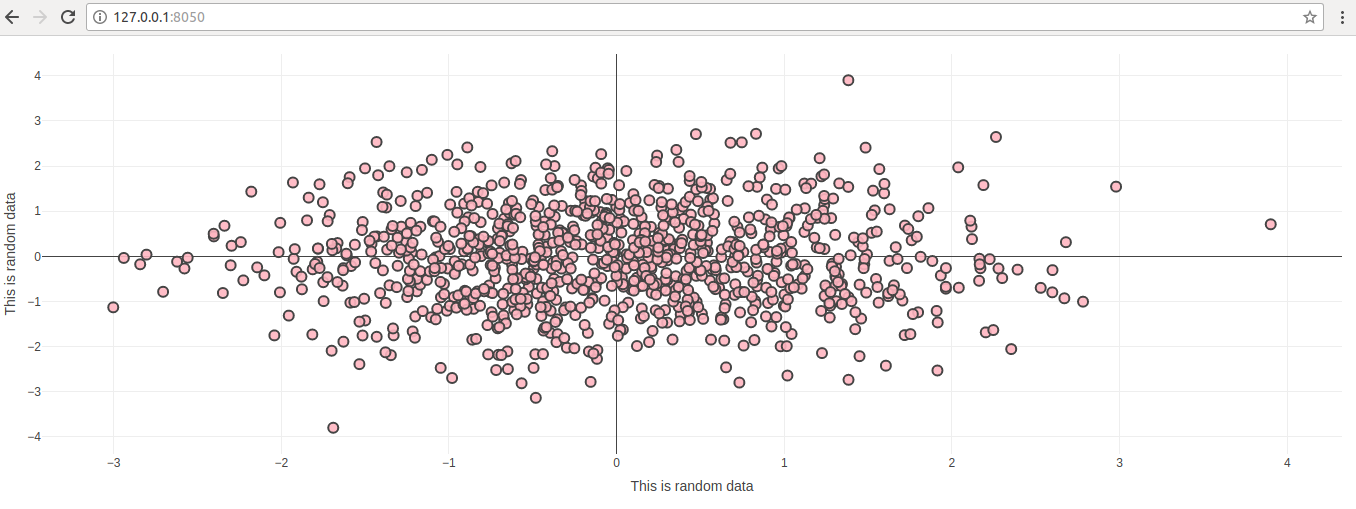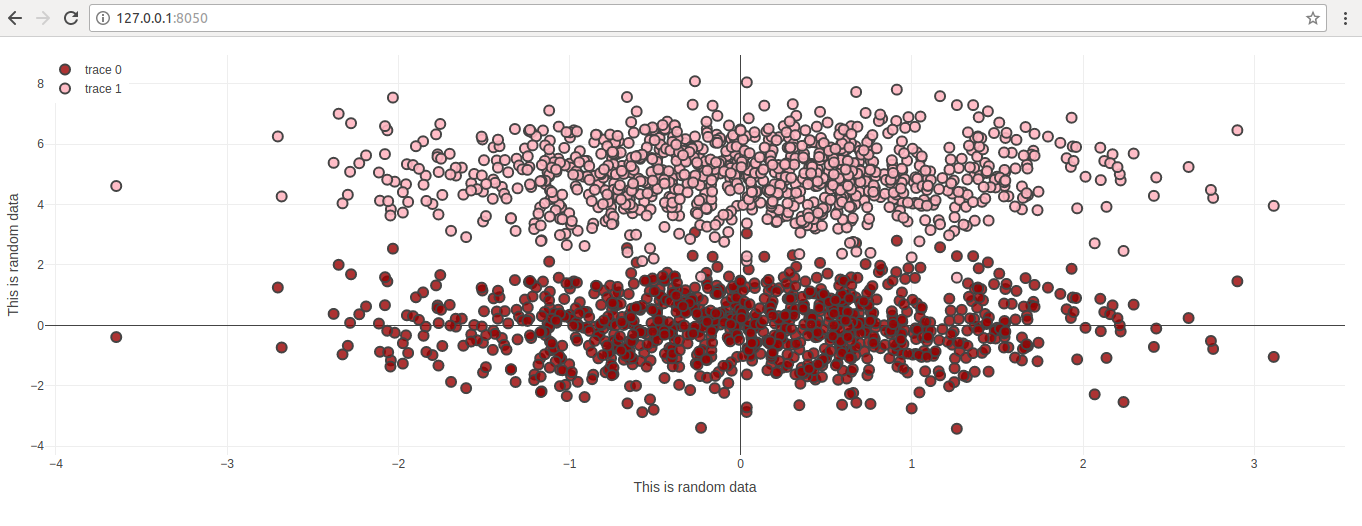# Customize Graphs Using Dash

In this article we will look at different ways of customizing of dash graphs. We will take an example of scatter plot. We will start with simple scatter plot and then build on top of it. Let us start with basic scatter plot which was referred in post Simple graphs using Dash

### Simple Scatter plot

``````
import dash
import dash_core_components as dcc
import dash_html_components as html
import pandas as pd
import plotly.graph_objs as go

app = dash.Dash()

import numpy as np

N = 1000
random_x = np.random.randn(N)
random_y = np.random.randn(N)
random_x2 = np.random.randn(N)
random_y2 = np.random.randn(N)

app.layout = html.Div([
dcc.Graph(
id='life-exp-vs-gdp',
figure={
'data': [
go.Scatter(
x = random_x,
y = random_y,
mode = 'markers',

)
],
}
)
])

if __name__ == '__main__':
app.run_server()
``````

### OutputNow let us add axis details and change color scheme of these dots on the scatter plot

``````
import dash
import dash_core_components as dcc
import dash_html_components as html
import pandas as pd
import plotly.graph_objs as go

app = dash.Dash()

import numpy as np

N = 1000
random_x = np.random.randn(N)
random_y = np.random.randn(N)
random_x2 = np.random.randn(N)
random_y2 = np.random.randn(N)

app.layout = html.Div([
dcc.Graph(
id='life-exp-vs-gdp',
figure={
'data': [
go.Scatter(
x = random_x,
y = random_y,
mode = 'markers',
marker = dict(
size = 10,
color = 'rgba(255, 182, 193, .9)',
line = dict(
width = 2,
)
)
)
],
'layout': go.Layout(
xaxis={'title': 'This is random data'},
yaxis={'title': 'This is random data'},
margin={'l': 40, 'b': 40, 't': 10, 'r': 10},
legend={'x': 0, 'y': 1},
hovermode='closest'
)
}
)
])

if __name__ == '__main__':
app.run_server()
``````

### Output### Scatter plot with multiple data sets

Here we have only one data set. Let us see how to add multiple data sets on the same scatter plot

``````
import dash
import dash_core_components as dcc
import dash_html_components as html
import pandas as pd
import plotly.graph_objs as go

app = dash.Dash()

import numpy as np

N = 1000
random_x = np.random.randn(N)
random_y = np.random.randn(N)

app.layout = html.Div([
dcc.Graph(
id='life-exp-vs-gdp',
figure={
'data': [
go.Scatter(
x = random_x,
y = random_y,
mode = 'markers',
marker = dict(
size = 10,
color = 'rgba(152, 0, 0, .8)',
line = dict(
width = 2,
)
)
),
go.Scatter(
x = random_x,
y = random_y+5,
mode = 'markers',
marker = dict(
size = 10,
color = 'rgba(255, 182, 193, .9)',
line = dict(
width = 2,
)
)
)
],
'layout': go.Layout(
xaxis={'title': 'This is random data'},
yaxis={'title': 'This is random data'},
margin={'l': 40, 'b': 40, 't': 10, 'r': 10},
legend={'x': 0, 'y': 1},
hovermode='closest'
)
}
)
])

if __name__ == '__main__':
app.run_server()
``````

### OutputYou can customize this further. Whatever you can do with plotly python module, you can do with Dash

CategoriesUncategorized